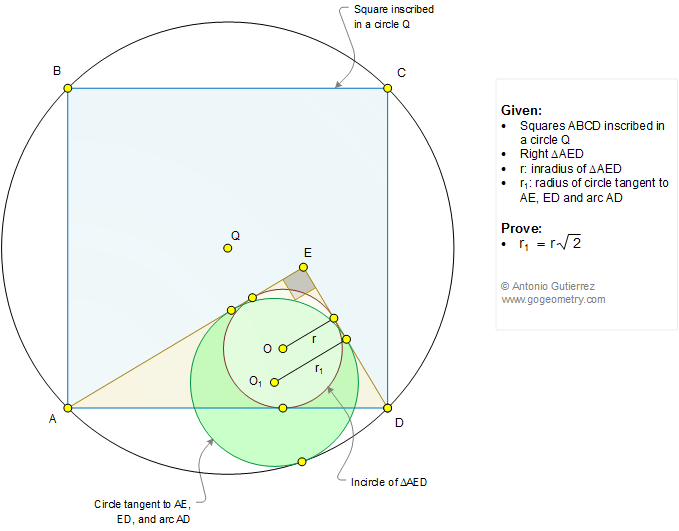# Geometry Problem 1292

### Elements: Square, Circle, Right Triangle, Arc, Inradius, Radius, Incircle, Measurement

The figure below shows a square ABCD inscribed in a circle of center Q and a right triangle AED (angle AED = 90 degrees) with inradius r. If r1 is the radius of a circle tangent to AE, ED, and arc AD, prove that r1 = r.sqrt(2).### Typography Art in Motion using Mobile Apps, iPad

Click on the figure below.